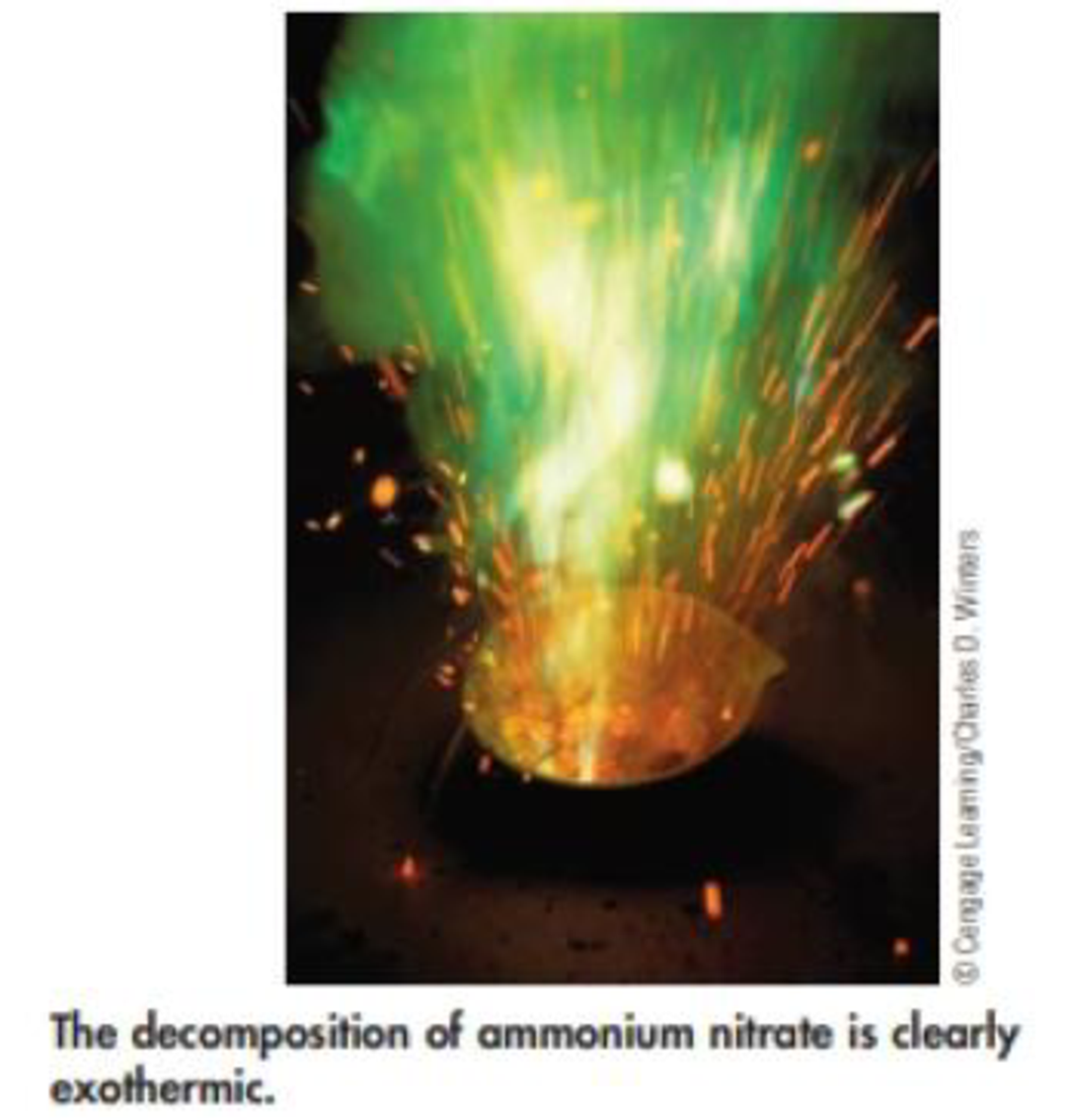Chapter 5, Problem 97IL

Chapter
Section
Textbook Problem

The value of ΔU for the decomposition of 7.647 g of ammonium nitrate can be measured in a bomb calorimeter. The reaction that occurs isNH4NO3(s) → N2O(g) + 2 H2O(g)The temperature of the calorimeter, which contains 415 g of water, increases from 18.90 °C to 20.72 °C. The heat capacity of the bomb is 155 J/K. What is the value of ΔU for this reaction, in kl/mol?Interpretation Introduction

Interpretation:

The change in internal energy has to be calculated.

Concept Introduction:

Heat energy required to raise the temperature of 1g of substance by 1K.Energy gained or lost can be calculated using the below equation.

q=C×m×ΔT

Where, q= energy gained or lost for a given mass of substance (m), C =specific heat capacity, ΔT= change in temperature.

Explanation

Given ΔT=1.82K

Specific heat capacity of water is 4.184J/gK

Heat capacity of calorimeter is 155J/K

Mass of the water is 415g

Using specific capacity of heat equation

q=C×m×ΔT, the values calculated.

Heat absorbed by the calorimeter =155J/K×1.82K =282J

Heat absorbed by water = 415g×4.184J/gK×1.82K = 3160J

Total heat absorbed =282J+3160J

Still sussing out bartleby?

Check out a sample textbook solution.

See a sample solution

The Solution to Your Study Problems

Bartleby provides explanations to thousands of textbook problems written by our experts, many with advanced degrees!

Get Started

What impact has recombinant DNA technology had on genetics and society?

Human Heredity: Principles and Issues (MindTap Course List)

What kind of spectrum does a neon sign produce?

Horizons: Exploring the Universe (MindTap Course List)

What is a metabolic pathway?

Chemistry for Today: General, Organic, and Biochemistry